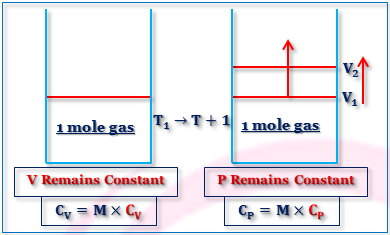## Articles by "PHYSICAL CHEMISTRY"

Showing posts with label PHYSICAL CHEMISTRY. Show all posts

### Chemical Equilibrium Questions and Answers

Given,
 (i) H2 (g) + 1/2 S2 (g) = H2S (g), KP1 (ii) 2H2 (g) + S2 (g) = 2H2S (g), KP2
Show that, KP2 = (KP1)2
For the first reaction, ΔG10 = - RT lnKP1
For the second reaction, ΔG20 = - RT lnKP2
Since, ΔG20 = 2ΔG10, therefore, it follows that,
- RT lnKP2 = - 2RT lnKP1
 ∴ KP2 = (KP1)2Equilibrium Constant
Use Gibbs - Helmholtz equation to derive the Vant Hoff reaction isochore. Under What condition do you expect a linear relationship between logk and 1/T ?
The Gibbs - Helmholtz equation is,
ΔG0 = ΔH0 + T[d(ΔG0)/dT]P
Zero superscript is indicating the stranded values.
or, - (ΔH0/T2 ) = -(ΔG0/T2 ) + 1/T[d(ΔG0)/dT]P
or, - (ΔH0/T2 ) = [d/dT(ΔG0/T)]P
Again Vant Hoff isotherm is,
- RT lnKP = ΔG0
or, - R lnKP = ΔG0/T
Differentiating with respect to T at constant P,
- R [dlnKP/dT]P = [d/dT(ΔG0/T)]P
Comparing the above two equation we have,
 dlnKP/dT = (ΔH0/T2
This is the Van't Hoff Equation isochore.
Greater the value of ΔH0, the faster the equilibrium constant(KP)changes with temperature(T), ΔH0 should remains constant for the linear plot of logKP vs 1/T.
How does the equilibrium constant for a reaction, 2A + 3B 4C + Heat, Change when (i) pressure is increases (ii) Temperature is decreases (iii) a catalyst added?
1. Equilibrium constant remains same when P is increases .
2. The reaction is exothermic, hence ΔH = (-)ve so the equilibrium constant is increased with decreases of temperature.
3. Equilibrium constant remains same though a catalyst is added.
4. ΔG0 = - RT lnKa but ΔG0 is not changed due to addition of catalyst as the latter does not participate in the reaction. The initial state and the final state of a chemical reaction remains same weather the catalyst is added or not.
Hence the equilibrium constant (Ka) remains unchanged.
For a reaction,2A + B 2C, ΔG0(500 K) = 2 KJ mol-1. Find the KP at 500 K for the reaction A + ½B C.
ΔG0 (500 K) for the reaction,
A + ½B C
= (2 KJ mol-1)/2
= 1 KJ mol-1
The relation is, ΔG0 = - RT lnKP
or, 1 = - (8.31 × 10-3) × (500) lnKP
or, lnKP = 1/(8.31 × 0.5)
= 0.2406
KP = 1.27
Justify or criticize the following: "The Equilibrium yield of products can not change if the equilibrium constant is kept fixed."
This statement is not correct.
Equilibrium yield of the product is changed if pressure is changed (Δγ ≠ 0), if inert gas is added at constant P (Δγ ≠ 0), and any of the reacting component is added a depleted.
For example, N2 + 3 H2 2NH3
Equilibrium yield of NH3 is increased if P is increased though equilibrium constant kept fixed.
Justify or criticize the following: Heat of reaction is the same weather a catalyst is used or not.
H is a state function hence ΔH (heat of a reaction) does no change if initial state and final state of a Process is same.
A catalyst can not change the initial and final state of a chemical reaction, hence ΔH remains same weather a catalyst is used or not.
Therefore the statement is correct.

## Heat Capacity Of Gases:

Heat capacity of a substance is defined as the amount of heat required to rise of the temperature by one degree. Heat capacity per gram of substance is called specific heat and per mole called molar heat capacity.
Thus, Molar heat capacity = Molar mass × Specific heat
CP = M × cp
CV = M × cv
Where CP and CV are the molar heat capacities at constant pressure and constant volume respectively. cp and cv are their specific heats.
The specific heat at constant pressure and constant volume are 0.125 and 0.075 Cal gm⁻¹K⁻¹ respectively. Calculate the molecular weight and atomicity of the gas. Name the gas if possible.
M = 40 and ⋎ = 1.66(mono-atomic), Ar(Argon).
For gases, there are two heat capacities at constant volume and constant pressure.
These are Represented as,
CV = (dq/dT)V = (dU/dT)V
CP = (dq/dT)P = (dU/dT)P + P(dV/dT)P
Difference in Heat Capacities of an Ideal Gas:
If the gas is assumed to be ideal, then,
PV = nRT and (dU/dT)V = (dU/dT)P
Again, P(dV/dT)P = nR
Thus for an ideal gas, PV = nRT; or, P(dV/dT)P = nR.
Again, CP = (dU/dT)P + P(dV/dT)P
or, CP = CV + {P×(nR/P)}
or, CP = CV +nR
For 1 mole ideal gas CP = Cv +R
Molar Heat capacities of Gases:Molar Heat Capacity Of Gases
From the above two descriptions, it is clear that CP and CV. Since for CP, some mechanical work is required as additional energy to absorbed for definite piston from volume V₁ to V₂. Thus , CP - CV = Mechanical Work = PdV = P(V₂-V₁) = PV₂-PV₁ = R(T+1) - RT = R
Again, CP = CV+R for 1 mole ideal gas.
Now let us find the expression of CV from the point of view of Kinetic Theory.
CV = Energy required to increase transnational kinetic energy of 1 mole gas for rise of 1° temperature + energy required to increase inter molecular energy of 1 mole gas for rise temperature of 1°.
Increase of transnational K.E. = (3/2)R(T+1) - (3/2)R = (3/2) R for 1 mole gas for 1° rise in temperature.
Mono-atomic Gases:
CV = (dU/dT)v = (3/2)R and CP = (5/2)R.
Thus γ = CP/CV = 5/3 ≃ 1.667
Poly-atomic Gases:
Linear  CV = (dU/dT)v = (3/2)R + R +(3N - 5)R
Non-linear  CV = (dU/dT)v = (3/2)R + (3/2)R +(3N - 6)R
Where N is the number of particles.
Molar Heat Capacities for Diatomic Molecules:
For diatomic molecule N = 2.
Thus CV = (3/2)R + R + R = (7/2)R and CP = (9/2)R.
∴ ⋎ = CP/CV = 9/7  ≃ 1.286
Molar Heat Capacities for Tri-atomic Molecules:
For tri-atomic molecule N = 3.
Thus CV(Linear) =

Name

Email *

Message *